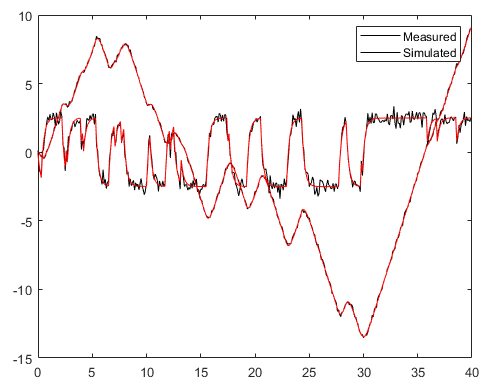Home > Projects/Reports > Matrix Assignment Example

# Matrix Assignment Example

Problem 8.7, But Instead of the Given System Use this One​​

x˙(t) =​​ 10-1-343254​​ x(t) +​​ 1-11u(t)

y(t)=​​ 300x(t)

Do the following​​

A). First, Verify Whether this System is Controllable and Observable​​

Solution:​​

First consider the system​​

x˙=​​ 10-1-343254​​ x+​​ 1-11u

y=​​ 300x

To check​​ the controllability and the​​ observable, consider the different matrix​​

First check system is controllable

x ˙= Ax +Bu

Y=Cx

Formula of Controllable matrix is​​

Pc=​​ B  AB  A2BAn-B

Pc=​​ 1-11 10-1-3432541-11 -1-5-5-93127-54029 1-11

Pc=​​  10-1-1-4-1311-15

Take detriment of​​ Pc=​​  10-1-1-4-1311-15

Det​​ Pc=70

The Determinant of the​​ Pc=70 ​​ is not Equal to Zero its Means the System is Controllable​​

Now check Observable

Po= CCACA2

Po= 300300*10-1-343254300*-1-13-15

Po= 30030-3-300

Take determent of​​ Po=30030-3-300

Po=0

So the System is not observable.

The final answer is that the given system is only Controllable.

B). Using the Poles Given in Problem 8.7, Compute the Corresponding State Feedback Vector K,​​ and Simulate The System With your Linear State Feedback Controller. Plot The​​

The linear feedback expression is​​

u=-Kx

Whereas K is the state feedback gain​​

K=​​ K1 K2

Calculate the matrix value​​

F-GK =​​ 10-1-343254-​​ 1-11K1 K2 K3

F-GK =​​ 1-K10-1-K1-3+K14 +K2 3+K32-K15-K2 4-K3-

To find the value of​​ K1 K2 K3 slove above matrix by row echelon form

F-GK=​​ -3430233600-2423

The value of the​​ K3= -2423

K2+K3=0

K2233-62423=0

K2= 6.2607.66

K2=0.817

-3K1 +4K2+3K3 =0

-3K1 +4(0.817)+3(-2423)=0

-3K1 +3.26-3.13=0

K1 =0.043

The Feedback of Controller is​​

K3= -2423

K2=0.817

K1 =0.043

c). What Arises When you Raise the Frequency of your Reference? Why?​​

If we increase the frequency of reference, the wavelength is associated with constant speed. The equation is c=f*λ,​​

As we know​​ that λ is the wavelength, and the f is the frequency​​

This is also happening when the source is moving away from the observer

Design a State Feedback Control System Satisfying the Following Specification:

(A). It Should Be Able to Tack A Train of Steps (Desired Azimuth Angle) That Alternates Between -1 And 1 Radians at A Frequency Of 1hz. The Settling Time Should Be at Most 0.25 Seconds. Show Your Tracking Performance Via A Plot of The Step Train Reference​​

x˙(t) =​​ 10-1-343254​​ x(t) +​​ 1-11u(t)

F=​​ 10-1-343254

G=​​ 1-11

The expression for peak​​ time

ts=πωd

0.25=​​ πωd

ωd= π0.25

ωd=12.56

Code:​​

tr =​​ Track of train:

​​ ​​ ​​ ​​ ​​ ​​ ​​​​ trainFcn: 'trainlm'

​​ ​​ ​​ ​​ ​​​​ trainParam: [1x1 struct]

​​ ​​ ​​ ​​ ​​​​ performFcn: 'mse'

​​ ​​ ​​​​ performParam: [1x1 struct]

​​ ​​ ​​ ​​ ​​ ​​ ​​​​ derivFcn: 'defaultderiv'

​​ ​​ ​​ ​​ ​​ ​​​​ divideFcn: 'dividerand'

​​ ​​ ​​ ​​ ​​​​ divideMode: 'sample'

​​ ​​ ​​ ​​​​ divideParam: [1x1 struct]

​​ ​​ ​​ ​​ ​​ ​​ ​​​​ trainInd: [1x176 double]

​​ ​​ ​​ ​​ ​​ ​​ ​​ ​​ ​​​​ valInd: [1x38 double]

​​ ​​ ​​ ​​ ​​ ​​ ​​ ​​​​ testInd: [1x38 double]

​​ ​​ ​​ ​​ ​​ ​​ ​​ ​​ ​​ ​​ ​​​​ stop: 'Validation stop.'

​​ ​​ ​​ ​​ ​​​​ num_epochs: 9

​​ ​​ ​​ ​​ ​​ ​​​​ trainMask: {[1x252 double]}

​​ ​​ ​​ ​​ ​​ ​​ ​​ ​​​​ valMask: {[1x252 double]}

​​  ​​ ​​ ​​ ​​ ​​ ​​​​ testMask: {[1x252 double]}

​​ ​​ ​​ ​​ ​​​​ best_epoch: 3

​​ ​​ ​​ ​​ ​​ ​​ ​​ ​​ ​​ ​​ ​​​​ goal: 0

​​ ​​ ​​ ​​ ​​ ​​ ​​ ​​ ​​​​ states: {1x8 cell}

​​ ​​ ​​ ​​ ​​ ​​ ​​ ​​ ​​ ​​​​ epoch: [0 1 2 3 4 5 6 7 8 9]

​​ ​​ ​​ ​​ ​​ ​​ ​​ ​​ ​​ ​​ ​​​​ time: [1x10 double]

​​ ​​ ​​ ​​ ​​ ​​ ​​ ​​ ​​ ​​ ​​​​ perf: [1x10 double]

​​ ​​ ​​ ​​ ​​ ​​ ​​ ​​ ​​ ​​​​ vperf: [1x10 double]

​​ ​​ ​​ ​​ ​​ ​​ ​​ ​​ ​​ ​​​​ tperf:​​ [1x10 double]

​​ ​​ ​​ ​​ ​​ ​​ ​​ ​​ ​​ ​​ ​​ ​​ ​​​​ mu: [1x10 double]

​​ ​​ ​​ ​​ ​​ ​​ ​​​​ gradient: [1x10 double]

​​ ​​ ​​ ​​ ​​ ​​ ​​​​ val_fail: [0 0 0 0 1 2 3 4 5 6]

​​ ​​ ​​ ​​ ​​ ​​​​ best_perf: 12.3078

​​ ​​ ​​ ​​ ​​​​ best_vperf: 16.6857

​​ ​​ ​​ ​​ ​​​​ best_tperf: 24.1796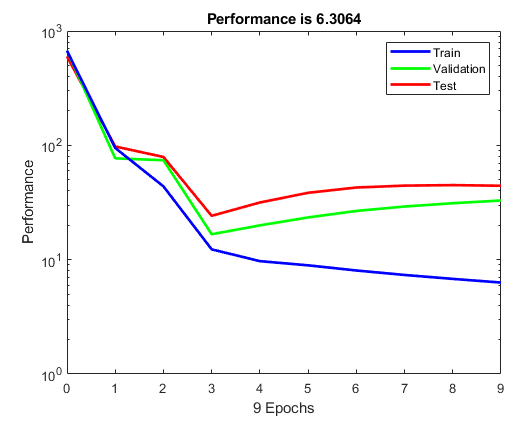B). Now Let the Azimuth Reference Be A Saw Tooth​​ Signal …., Demonstrate the Performance of Your Design by Providing A Corresponding Plot, and Make Sure you Show A Zoom of The Plot that Allows One to Verify That The Steady State Error Requirements Has Been Met​​

x = sawtooth(t)

x = sawtooth(t,xmax)

T = 10*(1/50);

Fs = 1;

dt = 1/Fs;

t = 0:dt:T-dt;

x = sawtooth(2*pi*50*t);

plot(t,x)

grid​​ on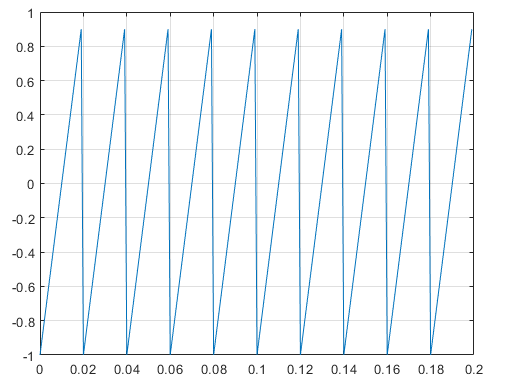## Project 2

Consider Problem 1 From Project 1, Where the Plant is given by

x˙(t) =​​ 10-1-343254​​ x(t) +​​ 1-11u(t)

y(t)=​​ 300x(t)

Do the following​​

A). Describe your overall Control design​​ solution​​

Control Design​​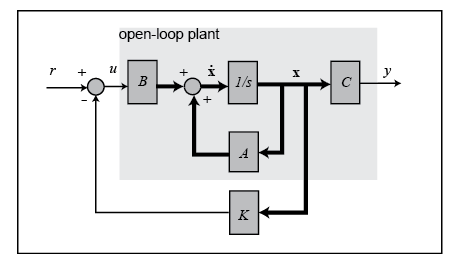For simplicity, let's assume the reference is zero,= 0. The input is then

U=-Kx​​

The state-space equations for the closed-loop feedback system are, therefore,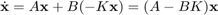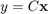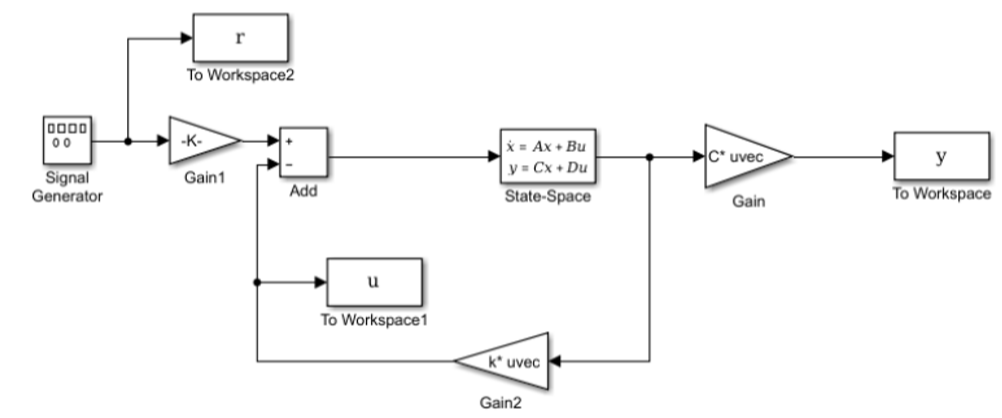The overall design solution is explained in project 1

B). Simulate the System with Your Control Solution. Demonstrate Your Design by Plotting in One Figure, The Real State and Its Estimates in Another The Real And Estimated Output Together With The Reference To Be Track. Show Two Full Periods of Yours Reference.​​

z = iddata(y,u,0.1,'Name','DC-motor');

[sys,x0] = n4sid(z,4);

[y,t,x] = lsim(sys,z.InputData,[],x0);

plot(t,z.OutputData,'k',t,y,'r')

legend('Measured','Simulated')